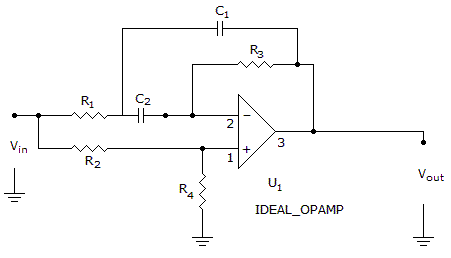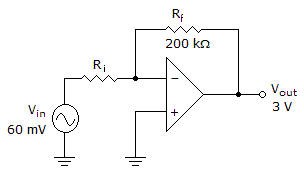# Electronics - Operational Amplifiers

Exercise : Operational Amplifiers - General Questions
6.
What is this circuit?a low-pass filter
a high-pass filter
a bandpass filter
a band-stop filter
Explanation:
No answer description is available. Let's discuss.

7.
A noninverting closed-loop op-amp circuit generally has a gain factor:
less than one
greater than one
of zero
equal to one
Explanation:
No answer description is available. Let's discuss.

8.
In order for an output to swing above and below a zero reference, the op-amp circuit requires:
a resistive feedback network
zero offset
a wide bandwidth
a negative and positive supply
Explanation:
No answer description is available. Let's discuss.

9.
Op-amps used as high- and low-pass filter circuits employ which configuration?
noninverting
comparator
open-loop
inverting
Explanation:
No answer description is available. Let's discuss.

10.Decreasing the gain in the given circuit could be achieved by

reducing the amplitude of the input voltage
increasing the value of the feedback resistor
increasing the value of the input resistor
removing the feedback resistor